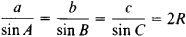# Sines, Law of

## Sines, Law of

a trigonometric theorem that establishes the relation between the sides a, b, and c of an arbitrary triangle and the sines of the angles A, B, and C opposite these sides. The law of sines states thatwhere R is the radius of the circumscribed circle.

Site: Follow: Share:
Open / Close